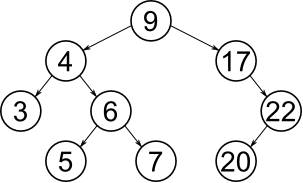# 8.1. `binary_tree` — Binary tree¶

A binary search tree consists of nodes, where each node has zero, one or two siblings. The left sibling has a lower value and the right sibling has a higher value than the parent.

Insert, delete and search operations all have the time complexity of O(log n).Test code: tst/collections/binary_tree/main.c

Test coverage: src/collections/binary_tree.c

Functions

int `binary_tree_init`(struct binary_tree_t *self_p)

Initialize given binary tree.

Return
zero(0) or negative error code.
Parameters
• `self_p` -

Binary tree.

int `binary_tree_insert`(struct binary_tree_t *self_p, struct binary_tree_node_t *node_p)

Insert given node into given binary tree.

There can not be two or more nodes in the tree with the same key. This function returns -1 if a node with the same key is already in the binary tree.

Return
zero(0) on success, -1 if a node with the same key is already in the binary tree, otherwise negative error code.
Parameters
• `self_p` -

Binary tree to insert the node into.

• `node_p` -

Node to insert.

int `binary_tree_delete`(struct binary_tree_t *self_p, int key)

Delete given node from given binary tree.

Return
zero(0) on success, -1 if the node was not found, otherwise negative error code.
Parameters
• `self_p` -

Binary tree to delete the node from.

• `key` -

Key of the node to delete.

struct binary_tree_node_t *`binary_tree_search`(struct binary_tree_t *self_p, int key)

Search the binary tree for the node with given key.

Return
Pointer to found node or NULL if a node with given key was not found in the tree.
Parameters
• `self_p` -

Binary tree to search in.

• `key` -

Key of the binary tree node to search for.

void `binary_tree_print`(struct binary_tree_t *self_p)

Print given binary tree.

Parameters
• `self_p` -

Binary tree to print.

struct `binary_tree_node_t`

Public Members

int `key`
int `height`
struct binary_tree_node_t *`left_p`
struct binary_tree_node_t *`right_p`
struct `binary_tree_t`

Public Members

struct binary_tree_node_t *`root_p`